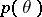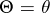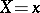# MediaWiki:Sidebar

Let <html>be a random parameter with an a priori density</html>, letbe a random result of observations and letbe the conditional density ofwhen; then the a posteriori distribution offor a given</html>, according to the Bayes formula, has the density